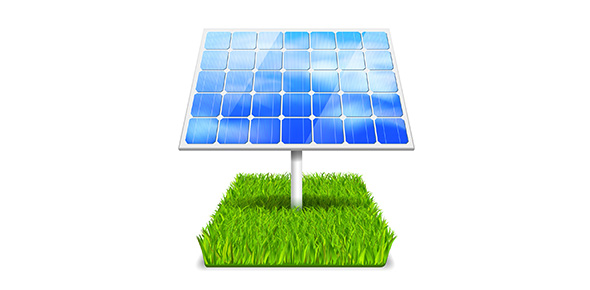# Corpus Christi Year 7 Energy Matters 2010 Quiz

25 Questions | Total Attempts: 593SettingsEnergy Matters Quiz- 25 Multiple Choice Questions. Students are to select the correct answer to the following questions.

• 1.
1.  Which group below are all liquids?
• A.

Water, salt, alcohol

• B.

Water, sugar, sea water

• C.

Alcohol, water and milk

• D.

Water, milk and flour

• 2.
2.  Substance which take the shape of their container, and take up a fixed amount of space are known as:
• A.

Solids

• B.

Liquids

• C.

Gases

• D.

Fluids

• 3.
As the temperature of a substance increases, its particles
• A.

Move faster

• B.

Move slower

• C.

Move at the same speed, but further apart

• D.

Stick together more

• 4.
A steel ball just fits through a steel ring at room temperature.The ball is then heated and now no longer fits through the ring. Choose which sentence below explains this using the particle model.
• A.

The particles in the ball start to move faster and the ball expands

• B.

The particles slow down and making the ball larger

• C.

The particles speed up and compress the ball

• D.

The particles speed up and as the ball gets very hot.

• 5.
Clouds are formed when:
• A.

Ice melts

• B.

Water evaporates

• C.

Water vapour condenses

• D.

Water freezes

• 6.
Diffusion occurs when:
• A.

A particles in a liquid are poured

• B.

A a container explodes

• C.

A gas moves around, taking up the space in its container

• D.

A solid or liquids turns into a gas

• 7.
When an object expands, its volume will-
• A.

Increase

• B.

Decrease

• C.

Stay the same size

• D.

A solid or liquid turns into a gas

• 8.
Which of the following is correct?
• A.

Only gases contracted when cooled

• B.

Both gases and solids contract when cooled

• C.

Solids, liquids and gases contracted when cooled

• D.

Non of the above- all substances expand when cooled

• 9.
The transfer of heat by passing of vibrations from particle to particle in a substance is called:
• A.

Reflection

• B.

Convection

• C.

Conduction

• D.

• 10.
What are the units of measurement for the volume of liquids:
• A.

Mm

• B.

ML3

• C.

Cm

• D.

ML

• 11.
The densities of solids, liquids and gases relate to-
• A.

How closely the particles are packed into a unit volume

• B.

The mass of the particles

• C.

How closely the particles are packed into a unit volume, as well as the mass of the particles

• D.

Particle arrangement

• 12.
Which state of matter can be easily compressed?
• A.

Solids

• B.

Liquids

• C.

Gases

• D.

Gases and liquids

• 13.
If a black and a white car are left in the sun, which one would be hotter and why?
• A.

White, absorption of heat

• B.

Black, absorption of heat

• C.

White, reflection

• D.

Black, reflection

• 14.
Water boils at which temperature:
• A.

0°C

• B.

100°C

• C.

50°C

• D.

75°C

• 15.
When a substance changes from a solid to a liquid, the change of state is called:
• A.

Melting

• B.

Evaporating

• C.

Condensation

• D.

Freezing

• 16.
When a substance changes from a liquid to a gas, the change of state is called:
• A.

Freezing

• B.

Evaporation

• C.

Condensation

• D.

Solidification

• 17.
Dry Ice is an example of sublimation. It changes state from:
• A.

Solid to a liquid

• B.

Liquid to a gas

• C.

Solid to a gas

• D.

Gas to a solid

• 18.
What three things can happen to radiation heat when it reaches an object?
• A.

Transmitted, absorbed, reflected

• B.

Insulated, transmitted, shrink

• C.

Decreased, absorbed, conducted

• D.

Transmitted, increased, insulated

• 19.
A clinical thermometer is used to take human temperature. The liquid used in clinical thermometers to measure temperature is
• A.

Alcohol

• B.

Mercury

• C.

Potassium

• D.

Milk

• 20.
Which group below has only properties of a solid?
• A.

Fixed shape, can be compressed, particles spread out

• B.

No fixed shape, can't be compressed, particles tightly together

• C.

Fixed shape, can't be compressed, particles tightly together

• D.

Changes shape, can be poured, particles tightly together

• 21.
A convection current is caused as warm particles move up and cooler particles move down. A convection current can occur in -
• A.

Solids, liquids and gases

• B.

Gases

• C.

Liquids

• D.

Liquids and gases

• 22.
Air is a mixture of gases. The gas that has the highest percentage component in the atmosphere is:
• A.

Nitrogen

• B.

Oxygen

• C.

Carbon Dioxide

• D.

Water Vapour

• 23.
Which of the following is NOT a change of state?
• A.

Precipitation

• B.

Water puddles disappearing on a hot day

• C.

Ice disappearing into a drink

• D.

Mist forming on a mirror when you have a shower

• 24.
An insulator:
• A.

Is a good conductor of heat

• B.

Is a poor conductor of heat

• C.

• D.

Is needed for a substance to change state

• 25.
When water is being heated by a Bunsen Burner, it begins to boil. Why does the temperature of the water not change even though more energy is being added by the Bunsen?
• A.

It is not hot enough

• B.

Energy is used to break bonds between particles

• C.

The thermometer cannot go any higher

• D.

Energy is used to create stronger bonds between particles

Related TopicsBack to top